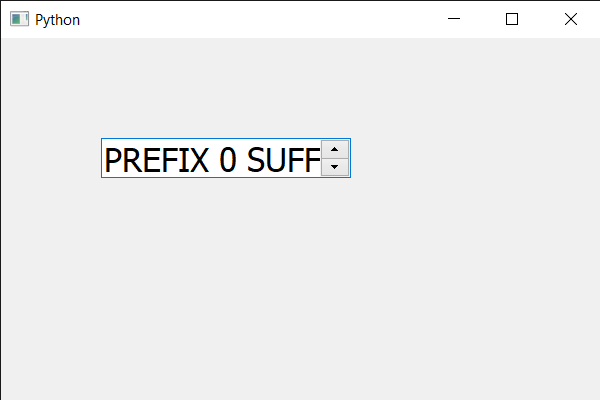# PyQt5 QSpinBox – Setting Point Size

• Last Updated : 22 May, 2020

In this article we will see how we can set the point size of the text in spin box. Changing the point size will change the size of the text, pixel and point are two different things some things point can have multiple pixels inside it. It is recommended to change point size for changing the size of the text.

In order to do this we use `setPointSize` method with the QFont object of the spin box

Attention geek! Strengthen your foundations with the Python Programming Foundation Course and learn the basics.

To begin with, your interview preparations Enhance your Data Structures concepts with the Python DS Course. And to begin with your Machine Learning Journey, join the Machine Learning - Basic Level Course

Syntax : font.setPointSize(n)

Argument : It takes integer as argument

Return : It returns None

Below is the implementation

 `# importing libraries``from` `PyQt5.QtWidgets ``import` `*` `from` `PyQt5 ``import` `QtCore, QtGui``from` `PyQt5.QtGui ``import` `*` `from` `PyQt5.QtCore ``import` `*` `import` `sys`` ` ` ` `class` `Window(QMainWindow):`` ` `    ``def` `__init__(``self``):``        ``super``().__init__()`` ` `        ``# setting title``        ``self``.setWindowTitle(``"Python "``)`` ` `        ``# setting geometry``        ``self``.setGeometry(``100``, ``100``, ``600``, ``400``)`` ` `        ``# calling method``        ``self``.UiComponents()`` ` `        ``# showing all the widgets``        ``self``.show()`` ` `        ``# method for widgets``    ``def` `UiComponents(``self``):``        ``# creating spin box``        ``self``.spin ``=` `QSpinBox(``self``)`` ` `        ``# setting geometry to spin box``        ``self``.spin.setGeometry(``100``, ``100``, ``250``, ``40``)`` ` `        ``# setting range to the spin box``        ``self``.spin.setRange(``0``, ``999999``)`` ` `        ``# setting prefix to spin``        ``self``.spin.setPrefix(``"PREFIX "``)`` ` `        ``# setting suffix to spin``        ``self``.spin.setSuffix(``" SUFFIX"``)`` ` `        ``# getting font of the spin box``        ``font ``=` `self``.spin.font()`` ` `        ``# setting point size``        ``font.setPointSize(``20``)`` ` `        ``# reassigning this font to the spin box``        ``self``.spin.setFont(font)`` ` `         ` `# create pyqt5 app``App ``=` `QApplication(sys.argv)`` ` `# create the instance of our Window``window ``=` `Window()`` ` `# start the app``sys.exit(App.``exec``())`

Output :My Personal Notes arrow_drop_up